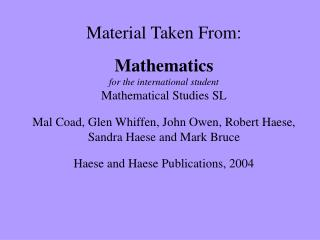DownloadDownload Presentation# Section 8e - Quadratic Equations

Download Presentation## Section 8e - Quadratic Equations

- - - - - - - - - - - - - - - - - - - - - - - - - - - E N D - - - - - - - - - - - - - - - - - - - - - - - - - - -
##### Presentation Transcript

1. Material Taken From:Mathematicsfor the international student Mathematical Studies SLMal Coad, Glen Whiffen, John Owen, Robert Haese, Sandra Haese and Mark BruceHaese and Haese Publications, 2004

2. Section 8e - Quadratic Equations

3. Solving Quadratic Equations ax2 + c = 0 isolate the squared term take the square root of both sides consider  solve

4. Solve for x 1) 2x2+ 1 = 15 • 2 – 3x2 = 8

5. Solve for x • (x – 3)2 = 16 • (x + 2)2 = 11

6. Zero Product Rule(a.k.a. Null Factor Law)

7. Solve for x • 3x(x – 5) = 0 • (x – 4)(3x + 7) = 0

8. Solving Quadratic Equations ax2 + c = 0 ax2 + bx = 0 isolate the squared term take the square root of both sides consider  solve set equal to zero factor out the GCF use the zero product property solve

9. Solve for x • x2= 3x • 2x2+ 8x = 0

10. Solving Quadratic Equations ax2 + c = 0 ax2 + bx = 0 ax2 + bx + c = 0 set equal to zero factor out the GCF, if possible factor use the zero product property solve isolate the squared term take the square root of both sides consider  solve set equal to zero factor out the GCF use the zero product property solve

11. Solve for x • x2= x + 30 • 3x2= 4x – 1

12. Solve for x • 12x2 – 5x = 2

13. Homework • Exercise 8E.1, pg255 #1acegi #2acei • Exercise 8E.2, pg 257 #1acfk • Exercise 8E.3, pg 257 #1ace #2acegj #3acegik #4ac #6aceg